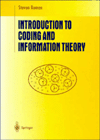##Introduction to Coding and Information Theory

### Springer-Verlag Undergraduate Texts in Mathematics

This book is intended to introduce coding theory and information theory to undergraduate students of mathematics and computer science. It begins with a review of probablity theory as applied to finite sample spaces and a general introduction to the nature and types of codes. The two subsequent chapters discuss information theory: efficiency of codes, the entropy of information sources, and Shannon's Noiseless Coding Theorem. The remaining three chapters deal with coding theory: communication channels, decoding in the presence of errors, the general theory of linear codes, and such specific codes as Hamming codes, the simplex codes, and many others.

1997, ISBN 0-387-94704-3, 323 pp., Hardcover